# AIM Ratios Rates and Conversions Ratios Rates and

• Slides: 8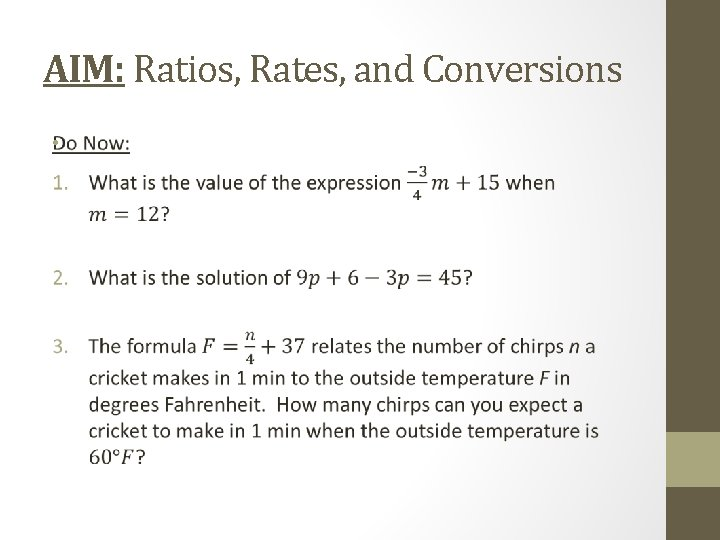AIM: Ratios, Rates, and Conversions •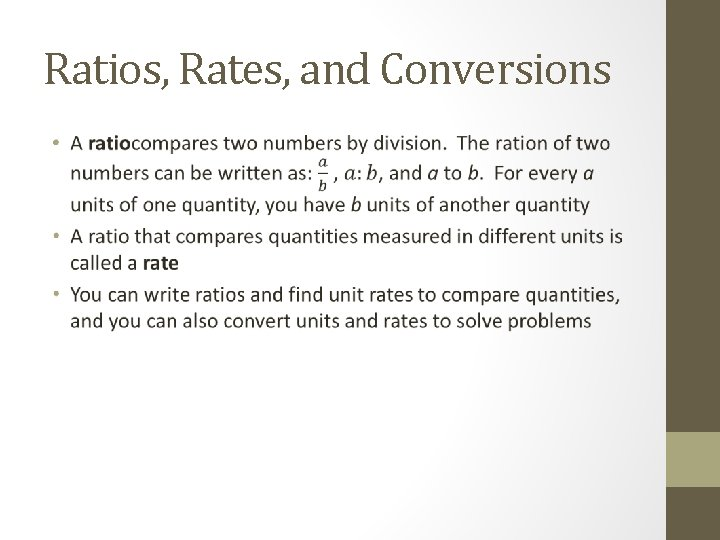Ratios, Rates, and Conversions •Ratios, Rates, and Conversions • Comparing Unit Rates You are shopping for T-shirts. Which store offers the best deal? Store A: \$25 for 2 shirts Store B: \$45 for 4 shirts Store C: \$30 for 3 shirts (HINT: write each price as a ratio, then write the ratio as a unit rate to compare?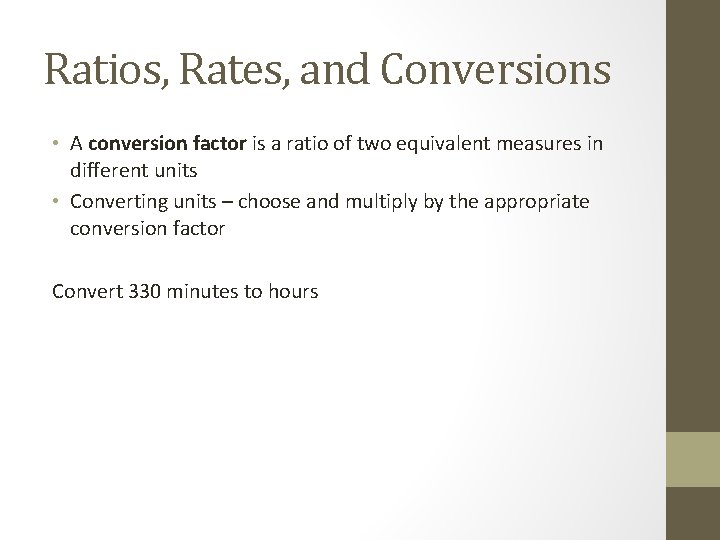Ratios, Rates, and Conversions • A conversion factor is a ratio of two equivalent measures in different units • Converting units – choose and multiply by the appropriate conversion factor Convert 330 minutes to hours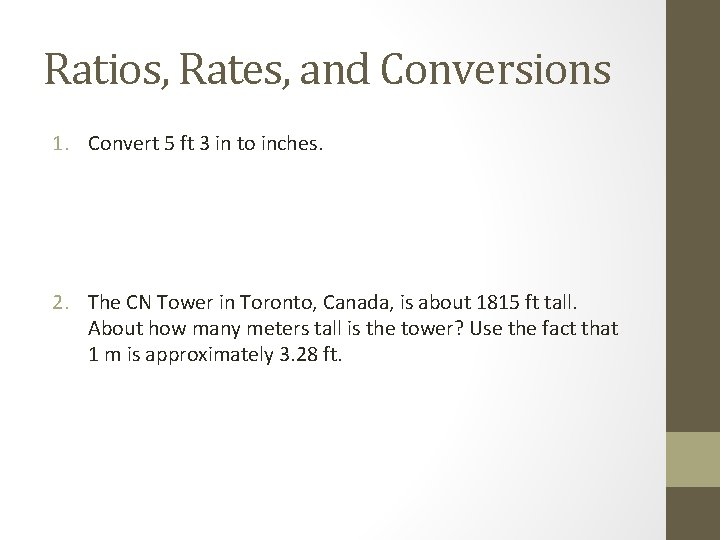Ratios, Rates, and Conversions 1. Convert 5 ft 3 in to inches. 2. The CN Tower in Toronto, Canada, is about 1815 ft tall. About how many meters tall is the tower? Use the fact that 1 m is approximately 3. 28 ft.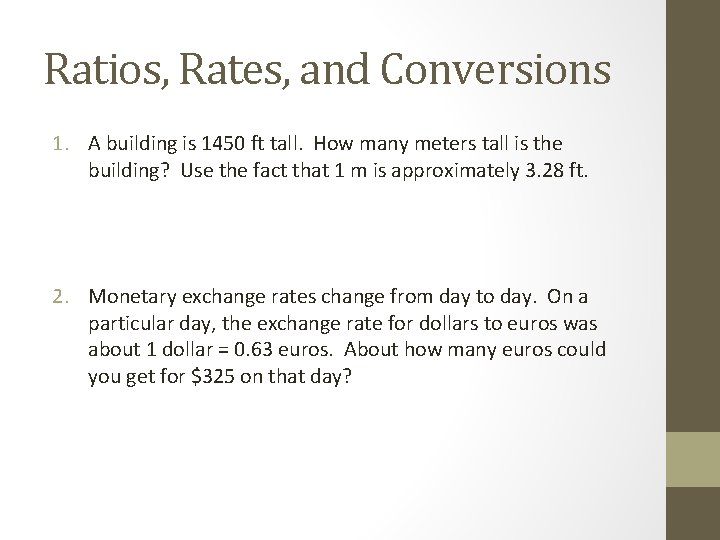Ratios, Rates, and Conversions 1. A building is 1450 ft tall. How many meters tall is the building? Use the fact that 1 m is approximately 3. 28 ft. 2. Monetary exchange rates change from day to day. On a particular day, the exchange rate for dollars to euros was about 1 dollar = 0. 63 euros. About how many euros could you get for \$325 on that day?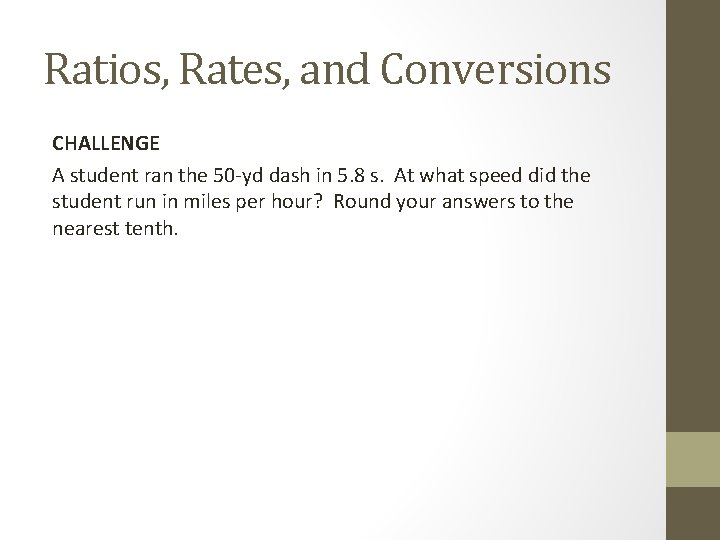Ratios, Rates, and Conversions CHALLENGE A student ran the 50 -yd dash in 5. 8 s. At what speed did the student run in miles per hour? Round your answers to the nearest tenth.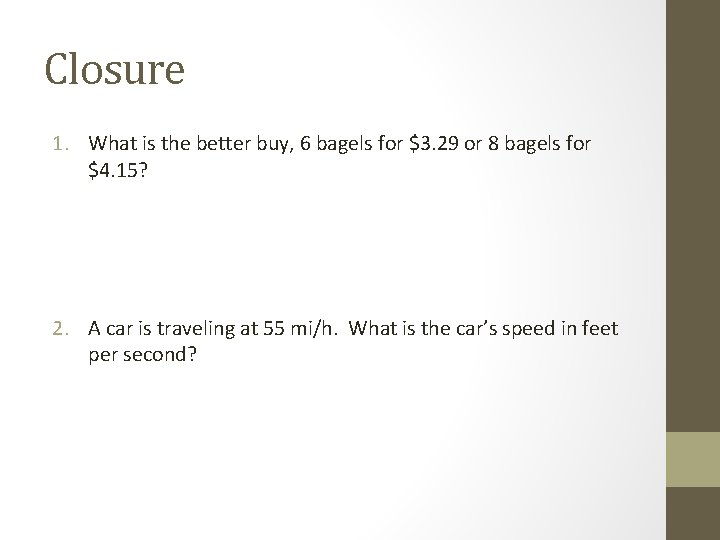Closure 1. What is the better buy, 6 bagels for \$3. 29 or 8 bagels for \$4. 15? 2. A car is traveling at 55 mi/h. What is the car’s speed in feet per second?